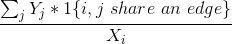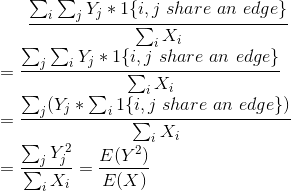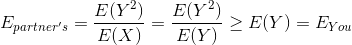It is not a relationship blog or anything even close to it. Just math. Pure applied math.

Assumptions:

• For this experiment we take in account only M2F relationships. I support sexual freedom to everyone, just the math is easier this way sooo…
• One sided relationship doesn’t count as a relationship. Sad. I know :(
• Self loving is also not a relationship. LOVE YOURSELF
• Number of guys and girls are considered equal as a fair assumption.
• A love triangle needs at-least one of the edge to be homosexual so not considered in our modelling.

Notations:

• X → Random Variable for number of relationship of girls
• Y → Random Variable for number of relationship of guys

Lemma 1: E(X) = E(Y)

Proof: If we model each individual as a node in graph, by our straight world assumption, the graph is bipartite and we can change all edge as directed going from a girl node to a guy node.

∑ X = Sum of out degrees of all node = Sum of in degrees = ∑ Y

Also, as ∑ 1{X = x} = ∑ 1{Y = y}, i.e. number of individuals in both gender is same, we have E(X) = E(Y).

#### Now for the real calculation,

For every girl i, X_i is the number of relationships she has been in. For every guy j, she has been with Y_j is his count. So, her perception for average girls a guy dates isi.e average of Y over all the guys she knows about i.e. X_i .

To calculate this quantity as an expectation, we need to sum this over every girl and every guy she has dated, i.e.Step 3 because if we sum all the instance i that has edges with j, it is same as the degree of j. Now, from the lemma E(X) = E(Y)The last part follows from the inequality E(X²) ≥ E(X)² and equality is in the case where variance of X is 0, surely not a case in dating world. As Y and X are interchangeable, the calculation for other general also follows.